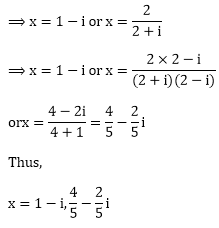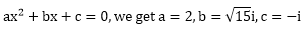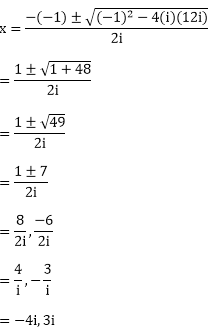#### Thank you for registering.

One of our academic counsellors will contact you within 1 working day.

Click to Chat

1800-5470-145

+91 7353221155

CART 0

• 0
MY CART (5)

Use Coupon: CART20 and get 20% off on all online Study Material

ITEM
DETAILS
MRP
DISCOUNT
FINAL PRICE
Total Price: Rs.

There are no items in this cart.
Continue Shopping

# Chapter 14: Quadratic Equations – Exercise 14.2

## Quadratic Equations – Exercise 14.2 – Q.1(i)

x2 + 10ix – 21 = 0

⟹ x2 + 10ix + 21i2 = 0              [∵  i2 = – 1]

⟹ x2 + 7ix + 3ix + 21i2 = 0

⟹ x(x + 7i) +3i(x + 7i) = 0

⟹ (x + 3i)(x + 7i) = 0

∴ x = – 3i, – 7i

## Quadratic Equations – Exercise 14.2 – Q.1(ii)

x2 + (1 – 2i)x – 1i = 0

⟹ x2 + x – 2i – 2i = 0

⟹ x(x + 1) – 2i(x + 1) = 0

⟹ (x – 2i)(x + 1) = 0

⟹ x = 2i, – 1

## Quadratic Equations – Exercise 14.2 – Q.1(iii)## Quadratic Equations – Exercise 14.2 – Q.1(iv)

6x- 17i x – 12 = 0

⟹ 6x- 17i x + 12i= 0              [∵ i= – 1]

⟹ 6x- 9ix – 8ix + 12i= 0

⟹3x(2x – 3i) – 4i(2x – 3i) = 0

⟹ (3x – 4i)(2x – 3i) = 0

⟹ x =4/3i or 3/2i

## Quadratic Equations – Exercise 14.2 – Q.2(i)## Quadratic Equations – Exercise 14.2 – Q.2(ii)

x2 – (5 – i)x + (18 + i) = 0

⟹ x- 5x – ix + 18 + i = 0

⟹ x- (3 – 4i)x – (2 + 3i)x + (18 + i) = 0

⟹ x(x – (3 – 4i)) – (2 + 3i)(x – (3 – 4i)) = 0

⟹ (x – (2 + 3i))(x – (3 – 4i)) = 0

⟹ x = 2 + 3i or 3 – 4i

## Quadratic Equations – Exercise 14.2 – Q.2(iii)

(2 + i)x- (5 – i)x + 2(1 – i) = 0

⟹ x [2 + i)x – 2] – (1 – i) [(2 + i)x – 2] = 0

⟹ [x -(1 – i) ][(2 + i) x – 2] = 0

either [x – (1 – i)] = 0 or [(2 + i) × – 2] = 0## Quadratic Equations – Exercise 14.2 – Q.2(iv)

x2 - (2 + i)x - (1 - 7i) = 0

⟹ x2 - (2 + i)x - (1 - 7i) = 0

⟹ x2 - (3 - i)x + (1 - 2i)x - (1 - 7i) = 0

⟹ x(x - (3 - i)) + (1 - 2i)(x - (3 - i)) = 0

⟹ [x + (1 - 2i)][x - (3 - i)] = 0

⟹ x =  – 1 + 2i, 3 –  i
⟹ x =  – 1 + 2i, 3 – i

## Quadratic Equations – Exercise 14.2 – Q.2(v)

ix- 4x - 4i = 0

⟹ ix2 + 4i2x + 4i3 = 0     [∵ i2 = – 1]

⟹ x2 + 4ix + 4i2 = 0

⟹ x2 + 2ix + 2ix + 4i2 = 0

⟹ x(x + 2i) + 2i(x + 2i) = 0

⟹ (x + 2i)(x + 2i)

∴  x = – 2i, – 2i

## Quadratic Equations – Exercise 14.2 – Q.2(vi)

x2 + 4ix – 4 = 0

⟹ x2 + 4ix + 4i2 = 0         [∵  i2 = – 1]

⟹ x2 + 2ix + 2ix + 4i2 = 0

⟹ x(x + 2i)+2i(x + 2i) = 0

⟹ (x + 2i)(x + 2i) = 0

⟹ x = – 2i, – 2i

## Quadratic Equations – Exercise 14.2 – Q.2(vii)Comparing the given equation with the general formSubstituting a and b in,⟹ – 15 + 8i = (a + bi)2

⟹ –15 + 8i = (a2- b+ 2abi

⟹a- b= – 15 and 2abi = 8i

Now (a+ b2)= (a- b2)+ 4a2b2

⟹(a+ b2)= (-15)+ 64 = 289

⟹ a+ b= 17

Solving a- b= – 15 and a+ b= 17, we get

a= 1 and b= 16

⟹ a = ± 1 and b = ± 4

⟹ a = ± 1 and b = ± 4

⟹ a = 1, b = 4 or a = – 1 b = – 4## Quadratic Equations – Exercise 14.2 – Q.2(viii)

x2 - x + (1 + i) = 0

x2 - x + (1 + i) = 0

x2 - ix -(1 - i)x + i(1 - i) = 0

(x - i)(x - (1 - i)) = 0

x = i, 1 - i

## Quadratic Equations – Exercise 14.2 – Q.2(ix)

We will apply discriminate rule on ax+ bx + c = 0,Now,

ix- x + 12i = 0## Quadratic Equations – Exercise 14.2 – Q.2(x)

We will apply discriminate rule on ax+ bx + c = 0,Now,